##### Algebra I For Dummies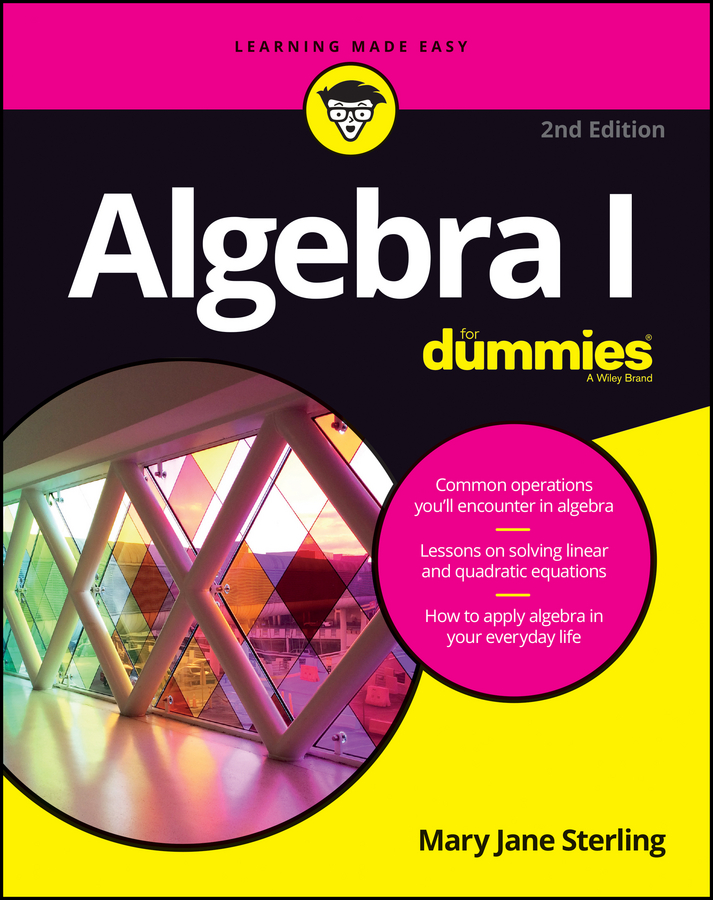The final outcomes of a binomial expansion depend on whether the original monomial had no coefficients or exponents (other than 1) of the variables. To find the expansion of binomials with the theorem in a basic situation, follow these steps:

1. Write out the binomial expansion by using the binomial theorem, substituting in for the variables where necessary.

If you need to find the entire expansion for a binomial, you can use the binomial theorem: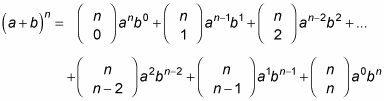For example, consider the problem (m + 2)4. According to the theorem, you should replace the letter a with m, the letter b with 2, and the exponent n with 4: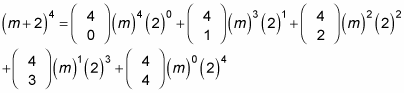The exponents of m begin at 4 and end at 0. Similarly, the exponents of 2 begin at 0 and end at 4. For each term, the sum of the exponents in the expansion is always 4.

2. Find the binomial coefficients.

This example uses the combinations formula to find the five coefficients, but you could use Pascal's triangle as a shortcut because the degree is so low (it wouldn't hurt you to write out five rows of Pascal's triangle — starting with 0 through 4).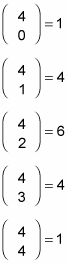You may have noticed that after you reach the middle of the expansion, the coefficients are a mirror image of the first half. This trick is a time-saver you can employ so you don't need to do all the calculations for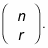3. Replace all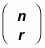4. with the coefficients from Step 2.

This step gives you

1(m)4(2)0 + 4(m)3(2)1 + 6(m)2(2)2 + 4(m)1(2)3 + 1(m)0(2)4

5. Raise the monomials to the powers specified for each term.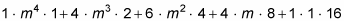6. Combine like terms and simplify.

m4 + 8m3 + 24m2 + 32m + 16

Notice that the coefficients you get in the final answer aren't the binomial coefficients you found in Step 1. This difference is because you must raise each monomial to a power (Step 4), and the constant in the original binomial changed the coefficient of each term.